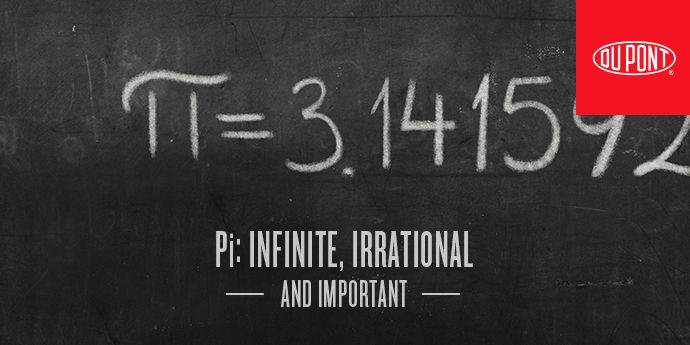# Pi: Infinite, Irrational & ImportantOn March 14, mathematicians, physicists, students and teachers around the world will observe Pi Day, a celebration of what has become the most famous number in all of mathematics. Pi is the ratio of the circumference of a circle to its diameter, roughly 3.14. This ratio is true of any circle, no matter how big or small.

Pi is an essential component for the formulas that calculate the area of a circle or the volume of a cylinder, which, granted, none of us do very often. But there’s more. Want to know how much material it will take to manufacture a basketball? Pi is involved (in calculating the surface of a sphere). Need to plot the frequency of sound waves? Pi is involved (in measuring sine waves). Need to calculate the orbit of a satellite? You’ll need Pi.

Pi also appears in dozens of formulas that are not well known outside of mathematical circles, but are nonetheless important. “Whenever there’s something circular going on, or any type of periodicity, Pi is lurking around somewhere,” says Joseph Mazur, author of Enlightening Symbols, a Short History of Mathematical Notation. “It appears in very unexpected places. If you relate the actual length of a river — following the curves — to its length end-to-end as the crow flies — you get a number very close to Pi.”

“Just about everyone’s used Pi in calculations at some point,” says Gayle Gibson, Director of DuPont Engineering. She also says that DuPont engineers use it every day to help improve products and meet customer needs.

### Ancient History

Pi has been around for a long time. There’s evidence that the Babylonians and ancient Egyptians were aware of Pi. The first formal approximation of its value was calculated by the famous Greek mathematician, Archimedes (287–212 BC).

Approximation is the key word here, as is clear from his method. First, he inscribed a regular polygon that fit as closely as possible inside a circle. Then he circumscribed another regular polygon that fit as closely as possible outside the same circle. Neither polygon would exactly represent the circumference of the circle, but he reasoned (correctly) that the circumference would lie somewhere in between. From this approach, he concluded that the value of Pi lies between 22/7 and 223/71.

With this method, it’s obvious that the more faces the two polygons have, the closer the approximation will be. Using two squares, for example, would not be nearly as accurate as using two octagons, and so on. With a geometrical approach, it’s possible to inscribe and circumscribe a circle with polygons that have many more faces — thousands, millions, even billions using a super computer — to get a closer and closer approximation.

Unfortunately for those who look to mathematics for the ultimate in precision, Pi is an irrational number. This means that, when expressed in decimals, it produces a string of digits that never ends, and never repeats any pattern in the unending string. With each decimal, you get closer to the truth, as it were, but you can never quite get there.

### What’s in a Name?

Until relatively recently, 3.14159265359 was known only by a Greek name (Pi) that when translated, meant “the quantity which, when the diameter is multiplied by it, yields the circumference.” William Jones, a little-known math teacher in England, first proposed the name Pi in 1706. It became internationally accepted when one of the most famous mathematicians of all time, Leonhard Euler, began using it in 1737. Some Pi fans believe that the Greek letter Pi was chosen because it is the first letter of perimeter, but this is not certain.

The idea of a holiday to celebrate the world’s most well-known numerical constant was “invented” by Larry Shaw, a now-retired physicist at The Exploratorium, a science museum in San Francisco. What began as a modest march followed by the consumption of fruit pies, has become a holiday celebrated worldwide.

Although it seems that Pi will continue to reign supreme in the realm of special numbers, it does have one important challenger. That’s Tau, which is the ratio of a circle’s circumference to its radius, roughly 6.28. The use of this alternative was first proposed in the now famous article, “Pi Is Wrong,” published by University of Utah math professor Bob Palais in 2001. Tauists, as they call themselves, celebrate Tau Day on June 28 (6.28, of course). They offer persuasive arguments related to both the teaching and practice of math and engineering, but it’s unlikely they’ll ever succeed in toppling Pi from its throne.

For now, we’ll continue to celebrate Pi as the king of special numbers.